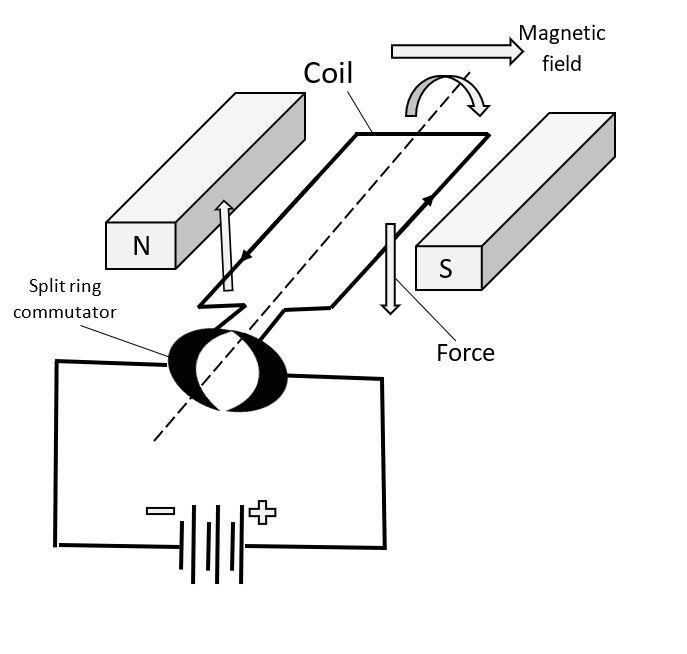QuestionAnswers

# State Faraday’s law of electromagnetic induction and Lenz’s law.

Hint: Faraday's law of electromagnetic induction which is also referred to as Faraday's law is a basic law of electromagnetism which predicts how a magnetic field will get interacted with an electric circuit in order to create an electromotive force (emf).$-N\Delta \phi \Delta t=emf$